# BREAK-EVEN ANALYSIS

5 de Jul de 2014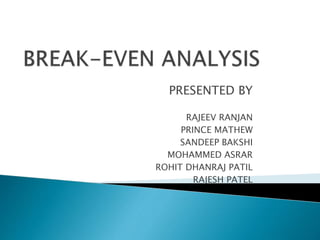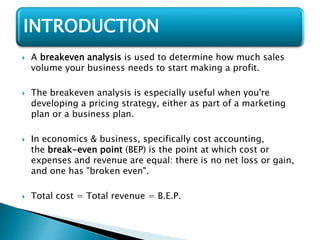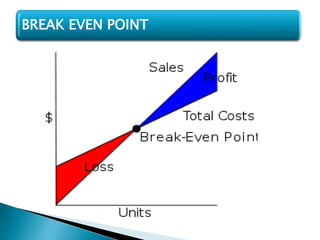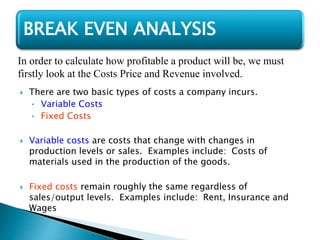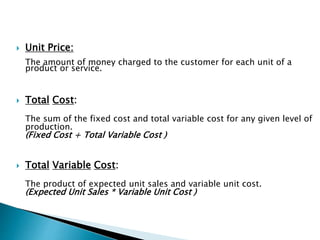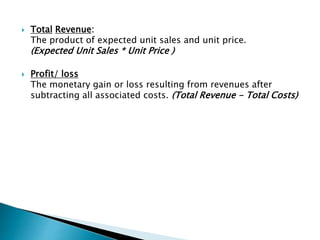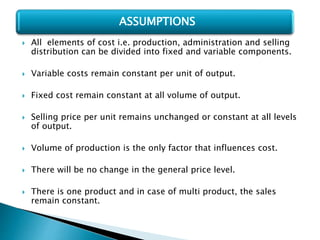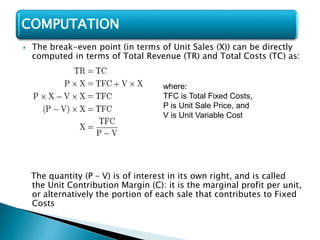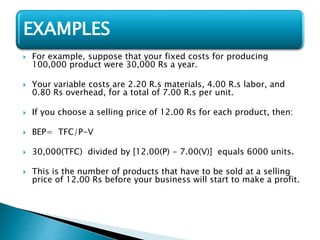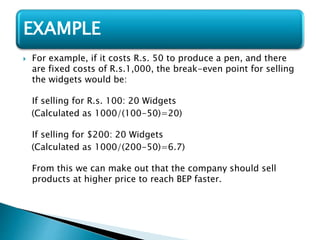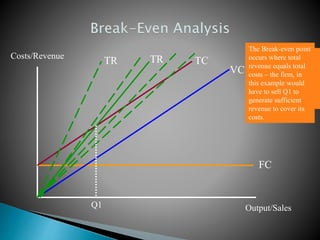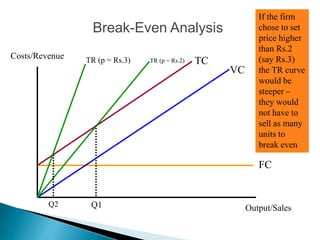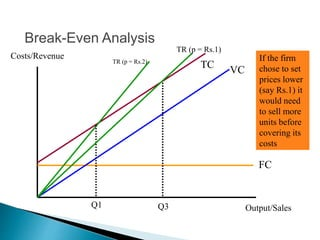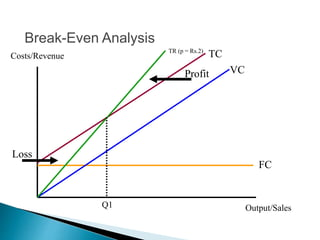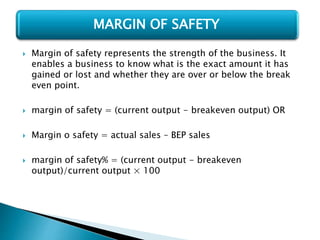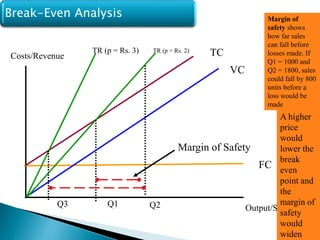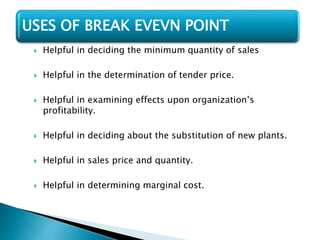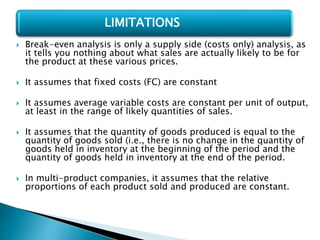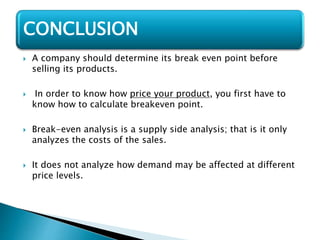1 de 19

### BREAK-EVEN ANALYSIS

• 1. PRESENTED BY RAJEEV RANJAN PRINCE MATHEW SANDEEP BAKSHI MOHAMMED ASRAR ROHIT DHANRAJ PATIL RAJESH PATEL
• 2.  A breakeven analysis is used to determine how much sales volume your business needs to start making a profit.  The breakeven analysis is especially useful when you're developing a pricing strategy, either as part of a marketing plan or a business plan.  In economics & business, specifically cost accounting, the break-even point (BEP) is the point at which cost or expenses and revenue are equal: there is no net loss or gain, and one has "broken even".  Total cost = Total revenue = B.E.P. INTRODUCTION
• 4.  There are two basic types of costs a company incurs. • Variable Costs • Fixed Costs  Variable costs are costs that change with changes in production levels or sales. Examples include: Costs of materials used in the production of the goods.  Fixed costs remain roughly the same regardless of sales/output levels. Examples include: Rent, Insurance and Wages BREAK EVEN ANALYSIS In order to calculate how profitable a product will be, we must firstly look at the Costs Price and Revenue involved.
• 5.  Unit Price: The amount of money charged to the customer for each unit of a product or service.  Total Cost: The sum of the fixed cost and total variable cost for any given level of production. (Fixed Cost + Total Variable Cost )  Total Variable Cost: The product of expected unit sales and variable unit cost. (Expected Unit Sales * Variable Unit Cost )
• 6.  Total Revenue: The product of expected unit sales and unit price. (Expected Unit Sales * Unit Price )  Profit/ loss The monetary gain or loss resulting from revenues after subtracting all associated costs. (Total Revenue - Total Costs)
• 7.  All elements of cost i.e. production, administration and selling distribution can be divided into fixed and variable components.  Variable costs remain constant per unit of output.  Fixed cost remain constant at all volume of output.  Selling price per unit remains unchanged or constant at all levels of output.  Volume of production is the only factor that influences cost.  There will be no change in the general price level.  There is one product and in case of multi product, the sales remain constant. ASSUMPTIONS
• 8.  The break-even point (in terms of Unit Sales (X)) can be directly computed in terms of Total Revenue (TR) and Total Costs (TC) as: The quantity (P – V) is of interest in its own right, and is called the Unit Contribution Margin (C): it is the marginal profit per unit, or alternatively the portion of each sale that contributes to Fixed Costs COMPUTATION where: TFC is Total Fixed Costs, P is Unit Sale Price, and V is Unit Variable Cost
• 9.  For example, suppose that your fixed costs for producing 100,000 product were 30,000 Rs a year.  Your variable costs are 2.20 R.s materials, 4.00 R.s labor, and 0.80 Rs overhead, for a total of 7.00 R.s per unit.  If you choose a selling price of 12.00 Rs for each product, then:  BEP= TFC/P-V  30,000(TFC) divided by [12.00(P) - 7.00(V)] equals 6000 units.  This is the number of products that have to be sold at a selling price of 12.00 Rs before your business will start to make a profit. EXAMPLES
• 10.  For example, if it costs R.s. 50 to produce a pen, and there are fixed costs of R.s.1,000, the break-even point for selling the widgets would be: If selling for R.s. 100: 20 Widgets (Calculated as 1000/(100-50)=20) If selling for \$200: 20 Widgets (Calculated as 1000/(200-50)=6.7) From this we can make out that the company should sell products at higher price to reach BEP faster. EXAMPLE
• 11. Costs/Revenue Output/Sales Initially a firm will incur fixed costs, these do not depend on output or sales. FC As output is generated, the firm will incur variable costs – these vary directly with the amount produced VC The total costs therefore (assuming accurate forecasts!) is the sum of FC+VC TC Total revenue is determined by the price charged and the quantity sold – again this will be determined by expected forecast sales initially. TR The lower the price, the less steep the total revenue curve. TR Q1 The Break-even point occurs where total revenue equals total costs – the firm, in this example would have to sell Q1 to generate sufficient revenue to cover its costs.
• 12. Break-Even Analysis Costs/Revenue Output/Sales FC VC TCTR (p = Rs.2) Q1 If the firm chose to set price higher than Rs.2 (say Rs.3) the TR curve would be steeper – they would not have to sell as many units to break even TR (p = Rs.3) Q2
• 13. Break-Even Analysis Costs/Revenue Output/Sales FC VCTCTR (p = Rs.2) Q1 If the firm chose to set prices lower (say Rs.1) it would need to sell more units before covering its costs TR (p = Rs.1) Q3
• 15.  Margin of safety represents the strength of the business. It enables a business to know what is the exact amount it has gained or lost and whether they are over or below the break even point.  margin of safety = (current output - breakeven output) OR  Margin o safety = actual sales – BEP sales  margin of safety% = (current output - breakeven output)/current output × 100 MARGIN OF SAFETY
• 16. Break-Even Analysis Costs/Revenue Output/Sales FC VC TCTR (p = Rs. 2) Q1 Q2 Margin of Safety Margin of safety shows how far sales can fall before losses made. If Q1 = 1000 and Q2 = 1800, sales could fall by 800 units before a loss would be made TR (p = Rs. 3) Q3 A higher price would lower the break even point and the margin of safety would widen
• 17.  Helpful in deciding the minimum quantity of sales  Helpful in the determination of tender price.  Helpful in examining effects upon organization’s profitability.  Helpful in deciding about the substitution of new plants.  Helpful in sales price and quantity.  Helpful in determining marginal cost. USES OF BREAK EVEVN POINT
• 18.  Break-even analysis is only a supply side (costs only) analysis, as it tells you nothing about what sales are actually likely to be for the product at these various prices.  It assumes that fixed costs (FC) are constant  It assumes average variable costs are constant per unit of output, at least in the range of likely quantities of sales.  It assumes that the quantity of goods produced is equal to the quantity of goods sold (i.e., there is no change in the quantity of goods held in inventory at the beginning of the period and the quantity of goods held in inventory at the end of the period.  In multi-product companies, it assumes that the relative proportions of each product sold and produced are constant. LIMITATIONS
• 19.  A company should determine its break even point before selling its products.  In order to know how price your product, you first have to know how to calculate breakeven point.  Break-even analysis is a supply side analysis; that is it only analyzes the costs of the sales.  It does not analyze how demand may be affected at different price levels. CONCLUSION